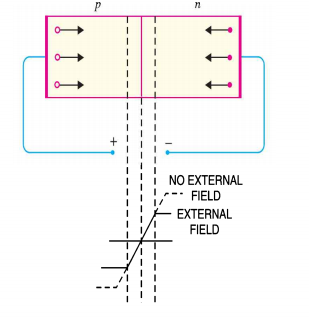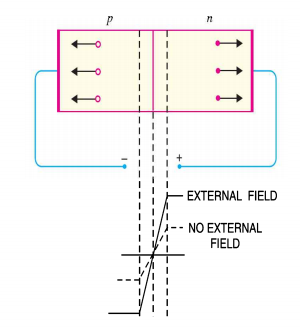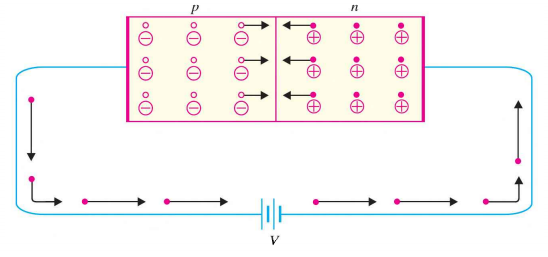# Forward & Reverse Biasing of pn Junction Diode

## Forward & Reverse Biasing of pn Junction Diode

In electronics, biasing means applying d.c. voltage to an electronics device to establish certain operating conditions.

In case of a p-n junction there are two bias conditions. Such as :

1. Forward biasing
2. Reverse biasing

### Forward Biasing of p-n Junction Diode

A p-n junction is said to be forward biased when the external d.c voltage applied in such a direction that it cancels the potential barrier, hence, permitting the current flow.

In order to forward bias a p-n junction , the positive terminal of the battery is connected to to the p-type and negative terminal of the battery is connected to the n-type as shown in the figure below.Fig.1: Forward Biasing of p-n Junction Diode

This applied forward potential establishes an electric field which acts against the field due to the potential barrier.

Hence, the resultant field is weakened and the barrier height at the junction is reduced as shown in the above figure.

Since the potential barrier voltage is very small (0.1 to 0.3 V), a small forward voltage is sufficient to completely eliminate the barrier.

Once the potential barrier is eliminated, junction resistance becomes almost zero and a low resistance path is established for the entire circuit. Thereby, current known as forward current flows in the circuit.

### Reverse Biasing of p-n Junction Diode

A pn junction is said to be reverse biased when the external d.c voltage applied to the junction is  in such a direction that it increases  the potential barrier .

In order to reverse bias a pn junction, the negative terminal of the battery is connected to the p-type and positive terminal is connected to the n-type as shown in the figure below.Fig.2:Reverse Biasing of p-n Junction Diode

This applied reverse voltage establishes an electric field which acts in the same direction as the field due to the potential barrier.

Hence, the resultant field at the junction is strengthened and the barrier height is increased as shown in the above figure.

The increased potential barrier prevents the flow of charge carriers across the junction.

Hence, a high resistance path is established for the entire circuit and therefore no current flows.

### Current Flow in a Forward Biased p-n junction

The figure below shows a forward biased p-n junction.Fig.3: Current Flow in a Forward Biased p-n Junction

When forward voltage is applied to a p-n junction, the free electrons in n-type move towards the junction, leaving behind positively charged atoms.

However, more electron from the negative terminal of the battery arrive and enter the n-region to take up their places.

As the free electrons reach the junction, they become valence electron.

As valence electron, they move through the holes in the p-region.

The valence electron move towards left in the p-region which is equivalent to the holes moving to right.

As the valence electron reach the left end of the crystal, they flow into the positive terminal of the battery.

### Summary

1. The free electrons from the negative terminal of the battery continue to pour into the n-region while the free electrons in the n-region move towards the junction.
2. The electrons travel through the n-region as free electrons hence, current in n-region is by free electrons.
3. When these electrons reach the junction, they combine with holes and become valence electrons.
4. As valence electron, they move through the holes in the p-region, hence, current int he p-region is by holes.
5. When these valence electrons reach the left end of the crystal, they flow into the positive terminal of the battery.